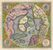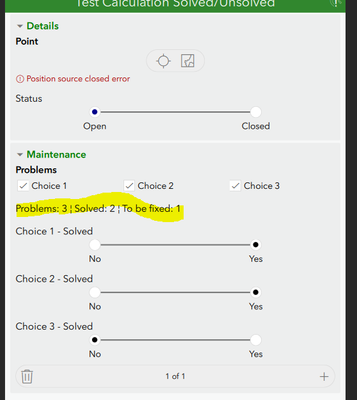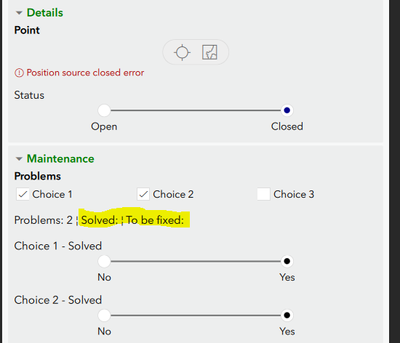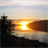# Help in Calculating Difference when a multiple selection is involved

600
10
01-18-2022 07:55 AMby
Regular Contributor

Hi,

I've created a calculation that counts how many problems are solved.

1. - Problems are first selected from a multiple selection question. Every problem, if selected is =1 in a hidden question. the sum of all of them gives me the "Problem = number"
2. When the problem is selected a question: "it has been solved" comes up: if answered yes, an hidden question collects =1 and they are summed up. Solved = number
3. A calculation Problems - Solved = to be fixed
4. this I know how many problems I have, how many fixed or not

It works perfectly if all my 3 problems are selected, if I select only one or two the calculation doesn't work: solved and to be fixed are empty.

this is a repeat table. By default all values are set to 0 {es.= if(selected(\${Problem},'Choice_1'),1,0)}, can't understand why the value Solved and To be fixed is empty

Thanks!Tags (2)
1 Solution

Accepted SolutionsbyMVP Honored Contributor

That makes more sense but it still wont work.

It is because all select one must be string.  So even though you give it a 0 and 1 it is really "0" and "1".  123 tries to help you when all three are filled out and gifts you the conversion.  This will prob not work at all on the web.

Best thing is to make a second set of if(value, 1, 0) and then count those just like you do for Choice_T.  I think it is the best way.  int("") is what is causing the non calc.

Other option that may work is to use coalesce("", 0) and put that inside the int.  Then it should give you 0 if it is sitting on blank.

Hope that helps

10 RepliesNew Contributor III

One way to fix that is to add two calculated fields (hidden) after each choices, one if the option is yes and one if no . for each answer set corresponding field to 1, then add a sum for each value solved yes or no. then you can use the sum fields for the label.byMVP Honored Contributor

My guess is you have  {es.= if(selected(\${Problem},'Choice_1'),1,0)}, outside the repeat.  Would help to see the form.by
Regular Contributor

all the calculations are inside the repeat.

This is my sample.

Thank you!byMVP Honored Contributor

I did not get a chance to test but I bet it is the bind.

For your 3 hidden counts like Choice1_T set the bind::type to int.  That way it is treated as a int in the form.  I know you have the esri bind there but that really just sets the service field and does not set the type while in the form.

I also see you use int later on most of them but you missed...

count_Problems   try setting that field bind type to int also.

So right here \${count_Problems} - int(\${count_Problems_Solved})  count _problems is still a string.

I think that should do it.

It is easier to just set the bind column vs using int all the time.  Hope that does it.by
Regular Contributor

I set all binds to int, and I fixed that calculation but still...same as before

I uploaded here the updated XLS, in case you have time to see it again.
Thank you for the hint. I have always overlooked that bind.byMVP Honored Contributor

Ah it was a simple mix up.  In count_problems_Solved you were adding up the Yes No questions and not the numbers field.  You were trying to add the strings.

You have int(\${Choice1}) +int(\${Choice2}) +int(\${Choice3})

when you want

int(\${Choice1_T}) +int(\${Choice2_T}) +int(\${Choice3_T})

Note you can take those int() out of there now since you set the bind field.

Also I would set the Choice1_T and the count solved fields to calculate vs hidden so they update better.

Works for me now I think you just got tripped up.by
Regular Contributor

Hi @DougBrowning thank you again!

I had a look at your suggestion but the problem is that I want to actually calculate : int(\${Choice1}) +int(\${Choice2}) +int(\${Choice3}) because these values count how many solved problems I have, instead int(\${Choice1_T}) +int(\${Choice2_T}) +int(\${Choice3_T}) calulates the original problems.

Then, the difference gives me the number of problemes that still need to be solved, don't know if it make sense.

\${Choice1} is a Yes or No question where no=0 and yes=1 and, I can't understand why, it sums up the values only when all 3 of them are selected (the default value is 0 and I believe here is the problem: this default value is not taken in consideration.)

If I choose all of the in the first question, then I have all of them are answered I have 0 +1 + 0 =1

If  I choose only one or two,  this doesn't work.byMVP Honored Contributor

That makes more sense but it still wont work.

It is because all select one must be string.  So even though you give it a 0 and 1 it is really "0" and "1".  123 tries to help you when all three are filled out and gifts you the conversion.  This will prob not work at all on the web.

Best thing is to make a second set of if(value, 1, 0) and then count those just like you do for Choice_T.  I think it is the best way.  int("") is what is causing the non calc.

Other option that may work is to use coalesce("", 0) and put that inside the int.  Then it should give you 0 if it is sitting on blank.

Hope that helpsby
Regular Contributor

@DougBrowning wrote:

Best thing is to make a second set of if(value, 1, 0) and then count those just like you do for Choice_T.  I think it is the best way.  int("") is what is causing the non calc.

Thank you!!! this  solution worked, and it is amazing.

Unfortunately, I realized that when I set the esri:parameter to allowUpdates=true the count goes back to NULL as it was before.

This is a pity because I need to review a previous survey and for some reason it doesn't pick up all the info, but I am still working on it. I have to see if there is a workaround.